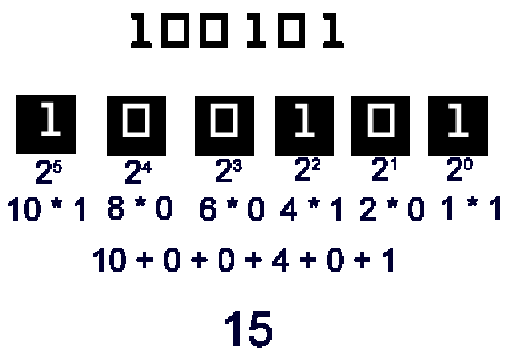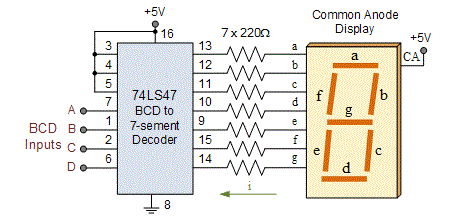# COMPUTER CODING SYSTEM

Many different coding systems are used to represent data today.

What is the importance of coding systems?

To represent numeric, alphabetic, and special characters in a computer’s internal storage and on magnetic media, we must use some sort of coding system.

In computers, the code is made up of fixed size groups of binary positions. Each binary position in a group is assigned a specific value; for example 8, 4, 2, or 1.

In this way, every character can be represented by a combination of bits that is different from any other combination.

Here we will learn about popular coding systems, used to represent data.

The coding also known as number systems include Binary, Octal, Hexadecimal, BCD and American Standard Code for Information Interchange (ASCII), Grey code and Excess three codes.

## BINARY CODING SYSTEM

Binary numbers were first described in Chandashutram written by Pingala in 100 BC.

Binary code was first introduced by the English mathematician and philosopher Eugene Paul Curtis during the 17th century.

Curtis was trying to find a system that converts logic’s verbal statements into a pure mathematical one. He created a system consisting of rows of zeros and ones.

During this time period, Curtis had not yet found a use for this system.Another Mathematician- George Boole developed Boolean algebra, based on binary.

After that, a graduate student, Claude Shannon noticed that the Boolean algebra he learned was similar to an electric circuit. Shannon wrote his thesis in 1937 and this was the starting point of the use of binary code in practical applications.

The binary numeral system, or base-2 number system, represents numeric values using two symbols: 0 and 1.

More specifically, the usual base-2 system is a positional notation with a radix of 2.

Because of its straightforward implementation in digital electronic circuitry using logic gates, the binary system is used internally by almost all modern computers.

Any number can be represented by any sequence of bits (binary digits), which in turn may be represented by any mechanism capable of being in two mutually exclusive states.

The numeric value represented in each case is dependent upon the value assigned to each symbol.

In a computer, the numeric values may be represented by two different voltages; on a magnetic disk, magnetic polarities may be used. A “positive”, “yes”, or “on” state is not necessarily equivalent to the numerical value of one; it depends on the architecture in use.

In keeping with customary representation of numerals using Arabic numerals, binary numbers are commonly written using the symbols 0 and 1. When written, binary numerals are often subscripted, prefixed or suffixed in order to indicate their base, or radix.

When spoken, binary numerals are usually read digit-by-digit, in order to distinguish them from decimal numerals. For example, the binary numeral 100 is pronounced one zero zero, rather than one hundred, to make its binary nature explicit, and for purposes of correctness.

Since the binary numeral 100 represents the value four, it would be confusing to refer to the numeral as one hundred (a word that represents a completely different value or amount).

Alternatively, the binary numeral 100 can be read out as “four” (the correct value), but this does not make its binary nature explicit.

### BINARY CODED DECIMAL

Many non-integral values, such as decimal 0.2, have an infinite place-value representation in binary but have a finite place-value in binary-coded decimal.

Consequently a system based on binary coded decimal representations of decimal fractions avoids errors representing and calculating such values.

In computing and electronic systems, binary-coded decimal (BCD) is a digital encoding method for numbers using decimal notation, with each decimal digit represented by its own binary sequence.

In BCD, a numeral is usually represented by four bits which, in general, represent the decimal range 0 through 9.

Other bit patterns are sometimes used for a sign or for other indications (e.g., error or overflow).

Uncompressed (or zoned) BCD consumes a byte for each represented numeral, whereas compressed (orpacked) BCD typically carries two numerals in a single byte by taking advantage of the fact that four bits will represent the full numeral range.BCD’s main virtue is ease of conversion between machine- and human-readable formats, as well as a more precise machine-format representation of decimal quantities.

As compared to typical binary formats, BCD’s principal drawbacks are a small increase in the complexity of the circuits needed to implement basic mathematical operations and less efficient usage of storage facilities.

BCD was used in many early decimal computers. Although BCD is not as widely used as in the past, decimal fixed-point and floating-point formats are still important and continue to be used in financial, commercial, and industrial computing, where subtle conversion and rounding errors that are inherent to floating point binary representations cannot be tolerated.

As described in the introduction, BCD takes advantage of the fact that any one decimal numeral can be represented by a four bit pattern.

As most computers store data in 8-bit bytes, it is possible to use one of the following methods to encode a BCD number:

• Uncompressed: each numeral is encoded into one byte, with four bits representing the numeral and the remaining bits having no significance.
• Packed: two numerals are encoded into a single byte, with one numeral in the least significant nibble (bits 0-3) and the other numeral in the most significant nibble (bits 4-7).

Hence the numerical range for one uncompressed BCD byte is zero through nine inclusive, whereas the range for one packed BCD is zero through ninety-nine inclusive.

To represent numbers larger than the range of a single byte any number of contiguous bytes may be used.

Note that the most significant nibble of the most significant byte is zero, implying that the number is in actuality 012345.

Also note how packed BCD is more efficient in storage usage as compared to uncompressed BCD; encoding the same number in uncompressed format would consume 100 percent more storage.

Shifting and masking operations are used to pack or unpack a packed BCD digit. Other logical operations are used to convert a numeral to its equivalent bit pattern or reverse the process.

Hence the numerical range for one uncompressed BCD byte is zero through nine inclusive, whereas the range for one packed BCD is zero through ninety-nine inclusive.

#### OCTAL CODING SYSTEM

The octal numeral system, or oct for short, is the base-8 number system, and uses the digits 0 to 7.

Numerals can be made from binary numerals by grouping consecutive binary digits into groups of three (starting from the right). For example, the binary representation for decimal 74 is 1001010, which can be grouped into (00)1 001 010 – so the octal representation is 112.

In decimal systems each decimal place is a base of 10.
In octal numerals each place is a power with base 8.

By performing the calculation above in the familiar decimal system we see why 112 in octal is equal to 64+8+2 = 74 in decimal.

In mathematics and computer science, hexadecimal (also base 16, or hex) is a positional numeral system with a radix, or base, of 16.

It uses sixteen distinct symbols, most often the symbols 0–9 to represent values zero to nine, and A, B, C, D, E, F (or alternatively a–f) to represent values ten to fifteen. For example, the hexadecimal number 2AF3 is equal, in decimal, to (2 × 163) + (10 × 162) + (15 × 161) + (3 × 160), or 10995.

Each hexadecimal digit represents four binary digits (bits), and the primary use of hexadecimal notation is a human-friendly representation of binary-coded values in computing and digital electronics.

One hexadecimal digit represents a nibble, which is half of an octet (8 bits). For example, byte values can range from 0 to 255 (decimal), but may be more conveniently represented as two hexadecimal digits in the range 00 to FF.

### ASCII (American standard code for information interchange)

The American Standard Code for Information Interchange (ASCII) was developed under the auspices of a committee of the American Standards Association, called the X3 committee, by its X3.2 (later X3L2) subcommittee, and later by that subcommittee’s X3.2.4 working group.

The ASA became the United States of America Standards Institute or USASI and ultimately the American National Standards Institute.

The American Standard Code for Information Interchange is a character-encoding scheme originally based on the English alphabet.

ASCII codes represent text in computers, communications equipment, and other devices that use text. Most modern character-encoding schemes are based on ASCII, though they support many additional characters.

ASCII developed from telegraphic codes. Its first commercial use was as a seven-bit teleprinter code promoted by Bell data services.

Work on the ASCII standard began on October 6, 1960, with the first meeting of the American Standards Association’s (ASA) X3.2 subcommittee.

The first edition of the standard was published during 1963, a major revision during 1967, and the most recent update during 1986.

Compared to earlier telegraph codes, the proposed Bell code and ASCII were both ordered for more convenient sorting (i.e., alphabetization) of lists and added features for devices other than teleprinters.

ASCII is the most common code in use today. One of the really nice things about ASCII is that all of the alpha characters are numbered sequentially; that is, 5 = A, 66 = B and so on until the end of the alphabet.

## CONCLUSION

These coding systems helps human to communicate with or through computers.

So the need for coding systems is clear. In this age of computers, it is essential. More than this many coding systems exists here now.

According to the increasing needs more and more developments are there in this field. As computer students we must understand about the updates in these systems.

Coding systems included in this are different from one another. But still there are some similarities. Knowing and understanding about coding systems is fun and essential.

error: Content is protected !!